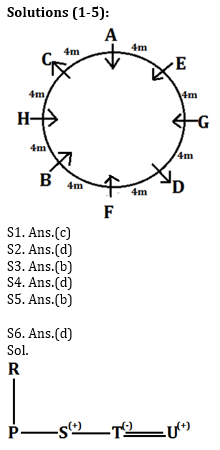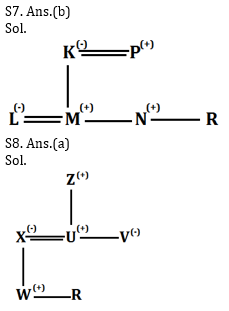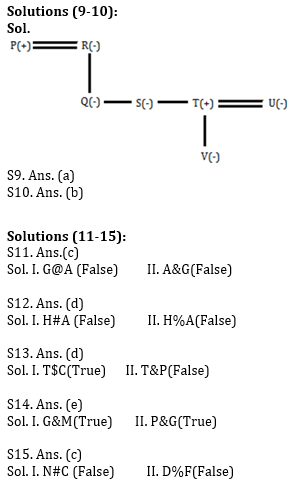Latest Banking jobs   »   Reasoning Ability Quiz for IBPS 2020...

# Reasoning Ability Quiz for IBPS 2020 Mains Exams- 5th December

Directions (1-5): Study the following information carefully and answer the questions given below.
Eight persons are sitting around a circular table which has a circumference of 32m. Each person is sitting at equal distance to each other. Some persons are facing outside while some are facing inside. Distance between F and C is 12m, C sits 8m right of E. G sits second to the right of F. Distance between G and B is 12m. D sits 8m right of B. A sits 16m right of F. Distance between E and H is 20m, when counted left of E. G is not an immediate neighbour of C. D sits 12m left of the one who sits 8m left of H. G is facing same direction of the one who sits 8m left C. D and G faces opposite direction to each other. C face outside the center and H sits immediate left of C.

Q1. Who among the following person sits 12m left of D?
(a) E
(b) F
(c) A
(d) G
(e) None of these

Q2. Who among the following sits opposite to the one who sits 4m right of G?
(a) F
(b) A
(c) C
(d) B
(e) None of these

Q3. Who among the following sits second to the right of A?
(a) F
(b) H
(c) G
(d) D
(e) None of these

Q4. How many persons are sitting between E and F, when counted right of E?
(a) Three
(b) One
(c) Two
(d) More than three
(e) None

Q5. Who among the following sits third to the left of H?
(a) C
(b) E
(c) D
(d) F
(e) None of these

Directions (6-8): In the following questions, the symbols #, &, @, * , \$, % and © are used with the following meanings as illustrated below. Study the following information and answer the given questions:
A@B- A is the child of B.
A©B- A is the parent of B
A%B- A is father-in-law of B
A&B- A is brother-in-law of B
A\$B- A is brother of B
A*B- A is wife of B
A#B- A is sister-in-law of B

Q6. If the expression “P@R©S\$T*U” is true, then how U is related to P?
(a) Father
(b) Sister
(c) Brother
(d) Brother-in-law
(e) None of these

Q7. If the expression “L*M@K©N\$R@P%L” is true, then how K is related to R?
(a) Father
(b) Mother
(c) Father-in-law
(d) Daughter-in-law
(e) None of these

Q8. If the expression “U\$V#X©W\$R, Z%X*U” is true, then which of the following is false?
(a) Z is grandmother of R
(b) X is mother of R
(c) U is brother of V
(d) V is aunt of W
(e) Both (c) and (a)

Directions (9-10): Study all the information carefully and answers the following questions given below:
There are 7 family members namely, P, Q, R, S, T, U and V in which 3 generation and 2 married couples. P is the father of S, whose sister in law is U. Q is the paternal aunt of V. T is the son of R but does not have any brother. T is the brother of S. V is not a male member of the family.

Q9. How is R related to V?
(a) Grandmother
(b) Grandfather
(c) Sister in law
(d) Aunt
(e) Can’t be determined

Q10. How many females are there in the family?
(a) Four
(b) Five
(c) Three
(d) Two
(e) None

Directions (11- 15): In the following questions, the symbols @, &, %, \$ and # are used with the following meaning as illustrated below:
‘P @ Q’ means ‘P is not smaller than Q’
‘P &Q’ means ‘P is neither greater than nor equal to Q’
‘P# Q’ means ‘P is neither greater than nor smaller than Q’
‘P \$ Q’ means ‘P is not greater than Q’
‘P % Q’ means ‘P is neither smaller than nor equal to Q’.
Now in each of the following questions assuming the given statements to be true, find which of the three conclusions follow and give answer accordingly.

Q11. Statement: A%B#C%D\$E; G@F%B
Conclusion: I. G@A II. A&G
(a) if only conclusion II is true.
(b) if only conclusion I is true.
(c) if neither conclusion I nor II is true.
(d) if either conclusion I or II is true.
(e) if both conclusions I and II are true.

Q12. Statement: R%T@J%A\$S#H; I%T
Conclusion: I. H#A II. H%A
(a) if both conclusion I and II are true.
(b) if only conclusion I is true.
(c) if neither conclusion I nor II is true.
(d) if either conclusion I or II is true.
(e) if only conclusion II is true.

Q13. Statement: C@A%R&P@W; A@N#T
Conclusion: I. T\$C II. T&P
(a) if only conclusion II is true.
(b) if either conclusion I or II is true.
(c) if neither conclusion I nor II is true.
(d) if only conclusion I is true.
(e) if both conclusions I and II are true.

Q14. Statement: P%T#D\$H@S; H%N;T#G; H&M
Conclusion: I.G&M II. P%G
(a) if only conclusion II is true.
(b) if only conclusion I is true.
(c) if neither conclusion I nor II is true.
(d) if either conclusion I or II is true.
(e) if both conclusions I and II are true.

Q15. Statement: N@V\$R#M%I#K; F&M; V%C; R%D
Conclusion: I. N#C II. D%F
(a) if only conclusion II is true.
(b) if either conclusion I or II is true.
(c) if neither conclusion I nor II is true.
(d) if only conclusion I is true.
(e) if both conclusions I and II are true.

Practice More Questions of Reasoning for Competitive Exams:

Reasoning for Competitive Exams
###### Reasoning Ability Quiz For IBPS 2020 Mains Exams- 3rd December
Prelims Quiz Study Plan for SBI & IBPS Exams 2020

SolutionsPractice with Crash Course and Online Test Series for IBPS Mains 2020:

Click Here to Register for Bank Exams 2020 Preparation Material#### Congratulations!Download Hindu Review of October 2021: Free PDF